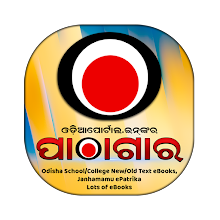## Recent PostsClass 1 to 10 All School eText Book, 80s-90s Old School Books, College Text Books, 250+ Janhamamu Magazine, Nijukti Khabar, Sishulekha Magazines Now Available on our new "Odia Pathagara" App.The following is the Revised Pattern of Evaluation & Distribution of Marks For H.S.C. Examination 2018 for Mathematics (MTH).

Mathematics (MTH)
ALGEBRA & GEOMETRY
Revised Pattern of Evaluation & Distribution of Marks For H.S.C. Examination 2018

PART - 1
OBJECTIVE (MCQ) TYPE  - TOTAL 50 MARKS

1. Twenty five (25) MCQ from Algebra - 1x25 = 25
2. Twenty five (25) MCQ from Geometry - 1x25=25

PART - 2
SUBJECTIVE TYPE  - TOTAL 50 MARKS

ALGEBRA
Q1. Two bit question with alternative choice carrying 5 (Five) marks each. - 5+5=10
Q2. Three bits question with alternative choice carrying 4 (four) marks each. - 4 + 4 + 4 = 12

GEOMETRY
Q3. One theorem and one construction with alternative choice carrying 5 (Five) marks each. - 5+5= 10
Q4. Rider and Trigonometry with alternative choice carrying 5 (Five) marks each. - 5 +5 = 10
Q5. Two bit question of mensuration carrying 4(Four) marks each. - 4 + 4 = 08

TOTAL MARKS: PART 1 (50) + PART 2 (50) = 100 MARKS